• 动态ReLU 在Pytorch上实现 。 例子 import torch.nn as nn from dyrelu import DyReluB class Model(nn.Module): def __init__(self): super(Model, self).__init__() self.conv1 = nn.Conv2d(3, 10, 5) self....
• 在GRU的基础上,对激活函数sigmoid,tanh,ReLU等性能进行了比较和研究,详细分析了几类激活函数的优缺点,提出了一种新的激活函数双曲正切线性单元(TLU)。实验证明:新的激活函数既能显著地加快深度神经网络的训练速度,又...
• 主要介绍了PyTorch之nn.ReLU与F.ReLU的区别介绍，具有很好的参考价值，希望对大家有所帮助。一起跟随小编过来看看吧
• m = nn.ReLU(inplace=True) input = torch.randn(7) print("输入处理前图片：") print(input) output = m(input) print("ReLU输出：") print(output) print("输出的尺度：") print(output.size()) print("输入处理后...
• 原地址：简易高效的LeakyReLu实现 代码如下： 我做了些改进，因为实在tensorflow中使用，就将原来的abs()函数替换成了tf.abs() import tensorflow as tf def LeakyRelu(x, leak=0.2, name=LeakyRelu): with tf....
• 在用Keras来实现CNN等一系列网络时，我们经常用ReLU作为激活函数，一般写法如下： from keras import layers from keras import models model = models.Sequential() model.add(layers.Conv2D(32, (3, 3), ...
• 在实现多层感知机代码实现中使用了ReLU激活函数： ReLU(x)=max(x,0)ReLU(x)=max(x,0)ReLU(x)=max(x,0) 形状大概是这样的 这里根据几个问题来进行回答解释 为什么要使用激活函数呢？ 简单来说激活函数的作用就是将...
• 基于 ReLU 和 Softmax 的简单深度神经网络 matlab 代码设计 本文以 matlab 为工具介绍下如何实现神经元激活函数为 ReLU 的深度神经网络 ReLU 函数的数学公式 很简单 ReLU(x)=max(x,0) 但其对 DNN 的贡献是巨大的若 ...
• ## ReLU

千次阅读 2019-11-10 14:46:01
ReLU Relu(rectified liner unit) 线性修正单元 ​ 在深度神经网络中，通常使用一种叫**修正线性单元(Rectified linear unit，ReLU）**作为神经元的激活函数。ReLU起源于神经科学的研究：2001年，Dayan、Abott从生物...
ReLU
Relu(rectified liner unit) 线性修正单元
​ 在深度神经网络中，通常使用一种叫**修正线性单元(Rectified linear unit，ReLU）**作为神经元的激活函数。ReLU起源于神经科学的研究：2001年，Dayan、Abott从生物学角度模拟出了脑神经元接受信号更精确的激活模型，如下图：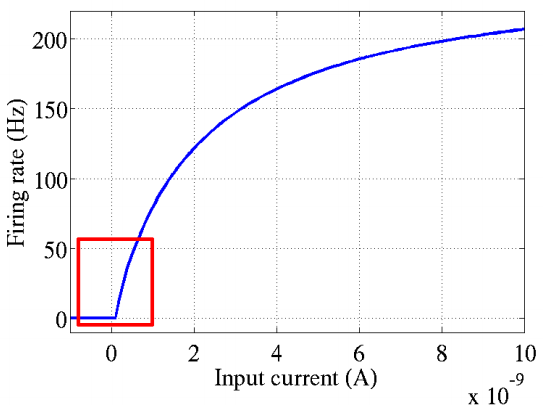其中横轴是时间(ms)，纵轴是神经元的放电速率(Firing Rate)。同年，Attwell等神经科学家通过研究大脑的能量消耗过程，推测神经元的工作方式具有稀疏性和分布性；2003年Lennie等神经科学家估测大脑同时被激活的神经元只有1~4%，这进一步表明了神经元的工作稀疏性。而对于ReLU函数而言，类似表现是如何体现的？其相比于其他线性函数(如purlin)和非线性函数(如sigmoid、双曲正切)又有何优势？
简单之美
首先，我们来看一下ReLU激活函数的形式，如下图：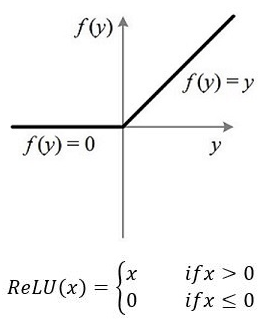​ 从上图不难看出，ReLU函数其实是分段线性函数，把所有的负值都变为
0，而正值不变，这种操作被成为单侧抑制。可别小看这个简单的操作，正因为有
了这单侧抑制，才使得神经网络中的神经元也具有了稀疏激活性。尤其体现在深
度神经网络模型(如CNN)中，
&&&当模型增加N层之后，理论上ReLU神经元的激活率将降低2的N次方倍。这里
或许有童鞋会问：ReLU的函数图像为什么一定要长这样？反过来，或者朝下延伸
行不行？其实还不一定要长这样。只要能起到单侧抑制的作用，
无论是镜面翻转还是180度翻转，最终神经元的输出也只是相当于加上了一个常数
项系数，并不影响模型的训练结果。之所以这样定，或许是为了契合生物学角
度，便于我们理解吧。
​ 那么问题来了：这种稀疏性有何作用？换句话说，我们为什么需要让神经元稀疏？不妨举栗子来说明。当看名侦探柯南的时候，我们可以根据故事情节进行思考和推理，这时用到的是我们的大脑左半球；而当看蒙面唱将时，我们可以跟着歌手一起哼唱，这时用到的则是我们的右半球。左半球侧重理性思维，而右半球侧重感性思维。也就是说，当我们在进行运算或者欣赏时，都会有一部分神经元处于激活或是抑制状态，可以说是各司其职。再比如，生病了去医院看病，检查报告里面上百项指标，但跟病情相关的通常只有那么几个。
与之类似，当训练一个深度分类模型的时候，和目标相关的特征往往也就那么几个，因此通过ReLU实现稀疏后的模型能够更好地挖掘相关特征，拟合训练数据。
ReLU VS sigmoid 、 线性函数
对于线性函数而言，ReLU的表达能力更强，尤其体现在深度网络中；
1时，预测值与真实值之间的误差每传播一层会衰减一次，如果在深层模型中使用sigmoid作为激活函数，这种现
象尤为明显，将导致模型收敛停滞不前。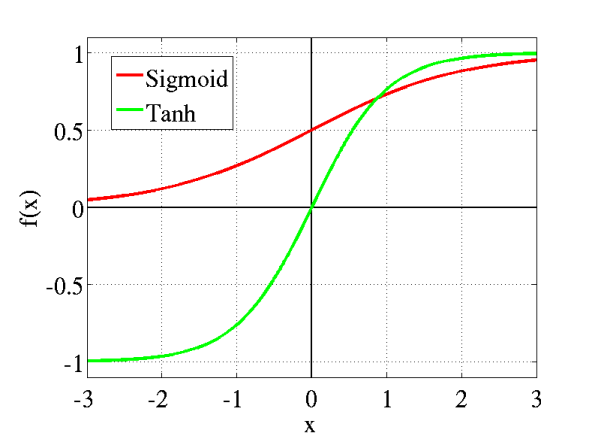s

i

g

m

o

i

d

=

1

1

−

e

−

z

sigmoid=\frac{1}{1-e^{-z}}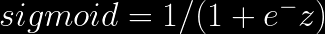sigmiod 函数 把我们计算的结果当成自变量放进去，让它的值域变为0~1之间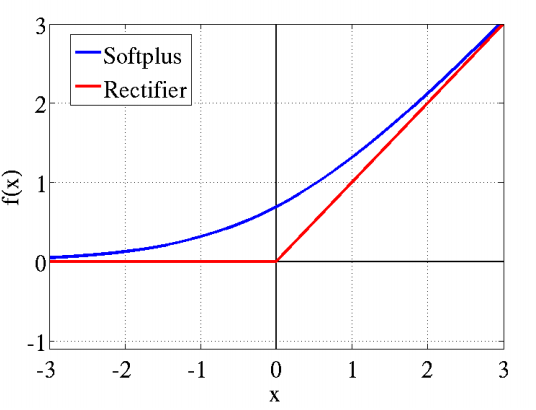ReLU与softplus函数与前图中的传统sigmoid系激活函数相比，主要变化有三点：
①单侧抑制 ②相对宽阔的兴奋边界 ③稀疏激活性（重点，可以看到红框里前端状态完全没有激活
@@@my thinking 不同的输入激活不同的区域，maybe就像我们大脑里不同的神经元一样专业分工，各司其职
ReLU 函数使不同的输入激活不同的区域，即稀疏激活性，总不能有个啥输入，全部细胞都一起来干活吧，杂七杂八的，容易把活干砸了。@@@
​
展开全文• 加标ReLU转换 ==== 用于训练和运行超高性能尖峰神经网络的转换代码。 引文 Diehl, P.U. and Neil, D. and Binas, J. and Cook, M. and Liu, S.C. and Pfeiffer, M. Fast-Classifying, High-Accuracy Spiking Deep ...MATLAB
• MNIST手写字识别 ReLU激活函数 规则化 识别率最高可达到97.5
• 关于Relu文章的理解翻译，原文见paper：Deep Sparse Rectifier Neural Networks
• ## Dynamic ReLU论文解读

千次阅读 2020-09-15 15:21:23
Dynamic ReLU论文解读。

其实一直在做论文阅读心得方面的工作，只是一直没有分享出来，这篇文章可以说是这个前沿论文解读系列的第一篇文章，希望能坚持下来。

简介
论文提出了动态线性修正单元（Dynamic Relu，下文简称 DY-ReLU），它能够依据输入动态调整对应分段函数，与 ReLU 及其静态变种相比，仅仅需要增加一些可以忽略不计的参数就可以带来大幅的性能提升，它可以无缝嵌入已有的主流模型中，在轻量级模型（如 MobileNetV2）上效果更加明显。
论文标题 Dynamic ReLU  论文地址 http://arxiv.org/abs/2003.10027  论文源码 https://github.com/Islanna/DynamicReLU
介绍
ReLU 在深度学习的发展中地位举足轻重，它简单而且高效，极大地提高了深度网络的性能，被很多 CV 任务的经典网络使用。不过 ReLU 及其变种（无参数的 leaky ReLU 和有参数的 PReLU）都是静态的，也就是说他们最终的参数都是固定的。那么自然会引发一个问题，能否根据输入的数据动态调整 ReLU 的参数呢？针对上述问题，论文提出了 DY-ReLU，它是一个分段函数

f

θ

(

x

)

(

x

)

f_{\boldsymbol{\theta}(\boldsymbol{x})}(\boldsymbol{x})

，其参数由超函数

θ

(

x

)

\boldsymbol{\theta {(x)}}

根据

x

x

计算得到。如上图所示，输入

x

x

在进入激活函数前分成两个流分别输入

θ

(

x

)

\boldsymbol{\theta {(x)}}

和

f

θ

(

x

)

(

x

)

f_{\boldsymbol{\theta}(\boldsymbol{x})}(\boldsymbol{x})

，前者用于获得激活函数的参数，后者用于获得激活函数的输出值。超函数

θ

(

x

)

\boldsymbol{\theta {(x)}}

能够编码输入

x

x

的各个维度（对卷积网络而言，这里指的就是通道，所以原文采用 c 来标记）的全局上下文信息来自适应激活函数

f

θ

(

x

)

(

x

)

f_{\boldsymbol{\theta}(\boldsymbol{x})}(\boldsymbol{x})

。
该设计能够在引入极少量的参数的情况下大大增强网络的表示能力，本文对于空间和通道上不同的共享机制设计了三种 DY-ReLU，分别是 DY-ReLU-A、DY-ReLU-B 以及 DY-ReLU-C。
Dynamic ReLU
定义原始的 ReLU 为

y

=

max

⁡

{

x

,

0

}

\boldsymbol{y}=\max \{\boldsymbol{x}, 0\}

，这是一个非常简单的分段函数。对于输入向量

x

x

的第

c

c

个通道的输入

x

c

x_c

，对应的激活函数可以记为

y

c

=

max

⁡

{

x

c

,

0

}

y_{c}=\max \left\{x_{c}, 0\right\}

。进而，ReLU 可以统一表示为带参分段线性函数

y

c

=

max

⁡

k

{

a

c

k

x

c

+

b

c

k

}

y_{c}=\max _{k}\left\{a_{c}^{k} x_{c}+b_{c}^{k}\right\}

，基于此提出下式动态 ReLU 来针对

x

=

{

x

c

}

\boldsymbol{x}=\left\{x_{c}\right\}

自适应

a

c

k

a_c^k

和

b

c

k

b_c^k

。

y

c

=

f

θ

(

x

)

(

x

c

)

=

max

⁡

1

≤

k

≤

K

{

a

c

k

(

x

)

x

c

+

b

c

k

(

x

)

}

y_{c}=f_{\boldsymbol{\theta}(\boldsymbol{x})}\left(x_{c}\right)=\max _{1 \leq k \leq K}\left\{a_{c}^{k}(\boldsymbol{x}) x_{c}+b_{c}^{k}(\boldsymbol{x})\right\}

系数

(

a

c

k

,

b

c

k

)

\left(a_{c}^{k}, b_{c}^{k}\right)

由超函数

θ

(

x

)

\boldsymbol{\theta (x)}

计算得到，具体如下，其中

K

K

为函数的数目，

C

C

为通道数目。且参数

(

a

c

k

,

b

c

k

)

\left(a_{c}^{k}, b_{c}^{k}\right)

不仅仅与

x

c

x_c

有关，还和

x

j

≠

c

x_{j \neq c}

有关。

[

a

1

1

,

…

,

a

C

1

,

…

,

a

1

K

,

…

,

a

C

K

,

b

1

1

,

…

,

b

C

1

,

…

,

b

1

K

,

…

,

b

C

K

]

T

=

θ

(

x

)

\left[a_{1}^{1}, \ldots, a_{C}^{1}, \ldots, a_{1}^{K}, \ldots, a_{C}^{K}, b_{1}^{1}, \ldots, b_{C}^{1}, \ldots, b_{1}^{K}, \ldots, b_{C}^{K}\right]^{T}=\boldsymbol{\theta}(\boldsymbol{x})

实现
DY-ReLU 的核心超函数

θ

(

x

)

\boldsymbol{\theta {(x)}}

的实现采用 SE 模块（SENet 提出的）实现，对于维度为

C

×

H

×

W

C \times H \times W

的张量输入，首先通过一个全局池化层压缩空间信息，然后经过两个中间夹着一个 ReLU 的全连接层，最后一个标准化层用于标准化输出的范围在

(

−

1

,

1

)

(-1,1)

之间（采用 sigmoid 函数）。该模块最终输出

2

K

C

2KC

个元素，分别是

a

1

:

C

1

:

K

a_{1: C}^{1: K}

和

b

1

:

C

1

:

K

b_{1: C}^{1: K}

的残差，记为

Δ

a

1

:

C

1

:

K

\Delta a_{1: C}^{1: K}

和

Δ

b

1

:

C

1

:

K

\Delta b_{1: C}^{1: K}

，最后的输出为初始值和残差的加权和，计算式如下。

a

c

k

(

x

)

=

α

k

+

λ

a

Δ

a

c

k

(

x

)

,

b

c

k

(

x

)

=

β

k

+

λ

b

Δ

b

c

k

(

x

)

a_{c}^{k}(\boldsymbol{x})=\alpha^{k}+\lambda_{a} \Delta a_{c}^{k}(\boldsymbol{x}), b_{c}^{k}(\boldsymbol{x})=\beta^{k}+\lambda_{b} \Delta b_{c}^{k}(\boldsymbol{x})

其中，

α

k

\alpha^k

和

β

k

\beta^k

分别为

a

c

k

a_c^k

和

b

c

k

b_c^k

的初始值，

λ

a

\lambda_a

和

λ

b

\lambda_b

为残差范围控制标量，也就是加的权。

α

k

\alpha^k

和

β

k

\beta^k

以及

λ

a

\lambda_a

、

λ

b

\lambda_b

都是超参数。若

K

=

2

K=2

，有

α

1

=

1

,

α

2

=

β

1

=

β

2

=

0

\alpha^{1}=1, \alpha^{2}=\beta^{1}=\beta^{2}=0

，这就是原始 ReLU。默认的

λ

a

\lambda_a

和

λ

b

\lambda_b

分别为 1.0 和 0.5。对于学习到不同的参数，DY-ReLU 会有不同的形式，它可以等价于 ReLU、Leaky ReLU 和 PReLU，也可以等价于 SE 模块或者 Maxout 算子，至于具体的形式依据输入而改变，是一种非常灵活的动态激活函数。
变种设计
主要提出三种不同的 DY-ReLU 设计，分别是 DY-ReLU-A、DY-ReLU-B 以及 DY-ReLU-C。DY-ReLU-A 空间和通道均共享，只会输出

2

K

2K

个参数，计算简单，表达能力较弱；DY-ReLU-B 仅空间上共享，输出

2

K

C

2KC

个参数；DY-ReLU-C 空间和通道均不共享，参数量极大。
实验结果
经过对比实验得出 DY-ReLU-B 更适合图像分类，DY-ReLU-C 更适合关键点检测任务，在几个典型网络上改用论文提出的 DY-ReLU，效果如下图，不难发现，在轻量级网络上突破较大。源码解析
下面是 DY-ReLU-B 的 Pytorch 实现。
import torch
import torch.nn as nn

class DyReLU(nn.Module):
def __init__(self, channels, reduction=4, k=2, conv_type='2d'):
super(DyReLU, self).__init__()
self.channels = channels
self.k = k
self.conv_type = conv_type
assert self.conv_type in ['1d', '2d']

self.fc1 = nn.Linear(channels, channels // reduction)
self.relu = nn.ReLU(inplace=True)
self.fc2 = nn.Linear(channels // reduction, 2*k)
self.sigmoid = nn.Sigmoid()

self.register_buffer('lambdas', torch.Tensor([1.]*k + [0.5]*k).float())
self.register_buffer('init_v', torch.Tensor([1.] + [0.]*(2*k - 1)).float())

def get_relu_coefs(self, x):
theta = torch.mean(x, axis=-1)
if self.conv_type == '2d':
theta = torch.mean(theta, axis=-1)
theta = self.fc1(theta)
theta = self.relu(theta)
theta = self.fc2(theta)
theta = 2 * self.sigmoid(theta) - 1
return theta

def forward(self, x):
raise NotImplementedError

class DyReLUB(DyReLU):
def __init__(self, channels, reduction=4, k=2, conv_type='2d'):
super(DyReLUB, self).__init__(channels, reduction, k, conv_type)
self.fc2 = nn.Linear(channels // reduction, 2*k*channels)

def forward(self, x):
assert x.shape == self.channels
theta = self.get_relu_coefs(x)

relu_coefs = theta.view(-1, self.channels, 2*self.k) * self.lambdas + self.init_v

if self.conv_type == '1d':
# BxCxL -> LxBxCx1
x_perm = x.permute(2, 0, 1).unsqueeze(-1)
output = x_perm * relu_coefs[:, :, :self.k] + relu_coefs[:, :, self.k:]
# LxBxCx2 -> BxCxL
result = torch.max(output, dim=-1).permute(1, 2, 0)

elif self.conv_type == '2d':
# BxCxHxW -> HxWxBxCx1
x_perm = x.permute(2, 3, 0, 1).unsqueeze(-1)
output = x_perm * relu_coefs[:, :, :self.k] + relu_coefs[:, :, self.k:]
# HxWxBxCx2 -> BxCxHxW
result = torch.max(output, dim=-1).permute(2, 3, 0, 1)

return result

这个结构和上文我所说的 SE 模块是大体对应的，目前支持一维和二维卷积，要想使用只需要像下面这样替换激活层即可（DY-ReLU 需要指定输入通道数目和卷积类型）。
import torch.nn as nn
from dyrelu import DyReluB

class Model(nn.Module):
def __init__(self):
super(Model, self).__init__()
self.conv1 = nn.Conv2d(3, 10, 5)
self.relu = DyReLUB(10, conv_type='2d')

def forward(self, x):
x = self.conv1(x)
x = self.relu(x)
return x


有空的话我会在 MobileNet 和 ResNet 上具体实验，看看实际效果是否如论文所述。

最近时间比较多，久违的填个坑，之前一直说要实验一下DyReLU的效果的，在Caltech256上进行对比实验，采用ResNet50和将部分ReLU换为DyReLU的ResNet5-dyrelu，在其他超参等配置完全一致的情况下，训练集表现和验证集表现如下图。（模型容量分别为370MB和414MB，略大了一些）如上图所示，加了DyReLU的模型训练更加平稳，收敛更快，损失降得更低。
总结
论文提出了 DY-ReLU，能够根据输入动态地调整激活函数，与 ReLU 及其变种对比，仅需额外的少量计算即可带来大幅的性能提升，能无缝嵌入到当前的主流模型中，是一个涨点利器。本质上，DY-ReLU 就是各种 ReLU 的数学归纳和拓展，这对后来激活函数的研究有指导意义。在ResNet50上进行对比实验的代码，开源于Github。
展开全文• DR: The premise for Leaky ReLU is that ReLU has a problem of being bounded on only one side and that any negative number has an output of 0, ‘killing’ the neuron. Leaky ReLU theoretically should pe....


TL;DR: The premise for Leaky ReLU is that ReLU has a problem of being bounded on only one side and that any negative number has an output of 0, ‘killing’ the neuron. Leaky ReLU theoretically should perform better, but is relatively the same in practice because the dead neuron problem is not common and can be remedied through other more mainstream methods.
TL; DR：泄漏ReLU的前提是ReLU存在仅在一侧受限制的问题，并且任何负数的输出均为0，从而“杀死”神经元。 从理论上讲，泄漏的ReLU的性能应该更好，但是在实践中相对相同，因为死亡的神经元问题并不常见，可以通过其他更主流的方法来解决。
The Rectified Linear Unit, abbreviated as ReLU, has shown incredible results when abundantly used in deep neural networks. Perhaps what is shocking about this success is that it is so simple: essentially a line bent at the origin such that the left half is y = 0 and the right is y = x.
当在深度神经网络中大量使用时，整流线性单位(缩写为ReLU)已显示出令人难以置信的结果。 可能令这一成功震惊的是，它是如此简单：本质上是一条在原点弯曲的线，使得左半部分为y = 0而右半部分为y = x 。
Graphed in Desmos. Equation is max(0, x).

Unlike curved activation functions like sigmoid or tanh, it doesn’t have a complex derivation or relation with the nature of probability — it is two lines. In fact, it can be difficult to see why ReLU works at all.
与诸如S型或tanh的曲线激活函数不同，它与概率的性质没有复杂的推导或关系-它是两条线。 实际上，很难理解ReLU为何起作用 。
We can peek into the answer by taking a look at feature maps of networks solving a two-circle problem over time with different activations.
我们可以通过查看随着时间的推移以不同的激活来解决两圆问题的网络的特征图来窥视答案。
Created by author.

ReLU draws the feature boundaries using corners, or junctions of lines, whereas a curved activation like tanh draws a curved envelope around the inside circle. The shape of the feature boundaries drawn by the network has heavy dependence on the choice of activation.
ReLU使用拐角或直线的交点绘制要素边界，而诸如tanh的弯曲激活则在内部圆周围绘制弯曲的包络线。 网络绘制的特征边界的形状在很大程度上取决于激活的选择。
Like all other activation functions, ReLU serves as a unit of a neuron, acting as some degree of freedom to the feature boundaries. However, it’s simple: the junction of two lines, rather than some trigonometric or exponential curve. Hence, the derivative is exceptionally easy to compute (if you’d even call it computing): 1 for the right half and 0 for the left half.
像所有其他激活功能一样，ReLU充当神经元的单元，对特征边界起一定程度的自由度。 但是，这很简单：两条线的交汇点，而不是某些三角或指数曲线。 因此，导数非常容易计算(如果您甚至称其为计算)：右半部分为1，左半部分为0。
Additionally, ReLU isn’t bounded on both sides; because the gradient is constant, there’s no need to worry of a vanishing gradient that plagues networks populated with bounded activations.
此外，ReLU并非两侧都有界。 由于梯度是恒定的，因此无需担心梯度消失的困扰，这些梯度困扰着充满激活作用的网络。
There is, however, a concern about the left half of ReLU, which yields 0 regardless of the input and perpetuates a malicious cycle. Say that prior to an input being passed through the activation, it is multiplied by a very large negative weight. The output of the neuron is 0, whose gradient is also 0.
但是，人们担心ReLU的左半部分，不管输入如何，该半部分都会产生0，并会持续一个恶意周期。 假设在输入通过激活之前，它乘以很大的负权重。 神经元的输出为0，其梯度也为0。
This gives the network essentially no information about the state of the weight in relation to the loss. Perhaps a weight of -3 is closer to performing better than -100,000; but the network wouldn’t know since the output for both is 0. Therefore, the weight is never updated because it is never updated — at this point, it is proclaimed to be ‘dead’.
这使得网络基本上没有有关重量状态的信息。 权重-3可能比-100,000更接近于表现； 但是网络不会知道，因为两者的输出均为0。因此，权重永远不会更新，因为它永远不会更新-在这一点上，它被称为“死”。
Note that bounded functions like sigmoid and tanh do not have this problem because their gradients are never equal to 0.
请注意，像Sigmoid和tanh这样的有界函数不会出现此问题，因为它们的梯度永远不会等于0。
Leaky ReLU uses the equation max(ax, x), opposed to max(0, x) for ReLU, where a is some small, preset parameter. This allows for some gradient to leak in the negative half of the function, which can provide more information to the network for all values of x.
泄漏的ReLU使用方程式max(ax, x) ，与ReLU的max(0, x)相反，其中a是一些小的预设参数。 这允许某些梯度在函数的负一半中泄漏，从而可以为x的所有值向网络提供更多信息。
a = 0.1. Graphed with Desmos.

Using Leaky ReLU makes sense, but empirically it has shown, at best, to have a slight advantage over ReLU. Most of the time, ReLU performs at the same level or even better than its alternative. Why is this the case?
使用泄漏的ReLU是有意义的，但从经验上看，它最多显示出比ReLU略有优势。 在大多数情况下，ReLU的性能与同类产品相同甚至更好。 为什么会这样呢？
Are dead neurons a significant threat?
死亡的神经元是否构成重大威胁？
Remember that there are always biases added to weights. If weights are initialized properly and biases act as supports, there should be a healthy gradient stream from the start. 请记住，权重总是存在偏差。 如果权重初始化正确，并且偏见作为支持，那么从一开始就应该有一个健康的渐变流。 Dead neurons form with overwhelmingly large negative weights. This can form two ways: a) the network is initialized poorly, or b) there is an exploding gradients problem that causes massive updates to weights. There are solutions to both. 死亡的神经元以极大的负重形成。 这可以形成两种方式：a)网络初始化不佳，或b)爆炸梯度问题导致权重的大量更新。 两者都有解决方案。 A dead neuron does not necessarily mean that the neuron’s output will be zero at testing; it all depends on the distribution of the inputs. This is, however, a small possibility. 死亡的神经元并不一定意味着在测试时神经元的输出将为零。 这一切都取决于投入的分配。 但是，这种可能性很小。 A dead neuron is not always permanently dead; the introduction of new training data can activate weights again through gradient descent. This is, again, a small possibility. 死亡的神经元并不总是永久死亡。 新训练数据的引入可以通过梯度下降再次激活权重。 再次，这是一个小可能性。 Given the size of modern neural nets, a few dead neurons has little to no impact. One can even argue that it acts like a fixed Dropout of sorts, restricting the network from passing inputs forward. 考虑到现代神经网络的规模，一些死亡的神经元几乎没有影响。 甚至可以说它像固定的Dropout，限制了网络将输入转发出去。
The primary issue — that is, massive updating of the weights, can be addressed through batch normalization, which smooths the loss space for more natural gradients; standard regulation, which prevents neurons from having weights that are too large in the first place; or even a properly set learning rate, which can reduce the step size of weights.
主要问题-权重的大量更新可以通过批量归一化解决 ，这可以平滑损失空间以实现更自然的梯度； 标准规则 ，首先防止神经元的权重过大； 甚至是正确设置的学习率 ，都可以减少权重的步长。
That being said, there are only two downsides towards using Leaky ReLU:
话虽如此，使用Leaky ReLU仅存在两个缺点：
The choice of a is not learnable like in Parametric ReLU, instead it must be set. Choosing the wrong value could do more detriment than good. 像在Parametric ReLU中一样，学习a的选择不是很容易，而是必须设置它。 选择错误的值可能弊大于利。 There’s not much usage of ReLU variants in the academic community, so most academics not directly studying activation functions will choose ReLU for an apples-to-apples comparison. ReLU变体在学术界没有太多用途，因此大多数不直接研究激活功能的学者都会选择ReLU进行苹果对苹果的比较。
In general, Leaky ReLU just hasn’t caught on that much in the deep learning community because there is no significant advantage. Granted, it has few reasons not to use, and some make the argument that a method with potential benefit and small downsides is reason enough for continuous usage.
通常，由于没有明显的优势，Leaky ReLU在深度学习社区中只是没有受到太大关注。 当然，它没有理由不使用，并且有些人认为，具有潜在利益和小的缺点的方法足以继续使用。
If you incorporate good practices into your network, like regularization (e.g. dropout, L1/L2), batch normalization, well-chosen optimizers with proper learning rates, etc., with most datasets dead neurons shouldn’t be much of a problem. But as some say — better safe than sorry.
如果您将良好做法(例如正则化(例如，辍学，L1 / L2)，批处理规范化，具有适当学习率的精心选择的优化器)整合到网络中，则对于大多数数据集而言，死去的神经元应该不是什么大问题。 但是正如某些人所说，安全要比后悔好。

翻译自: https://towardsdatascience.com/is-leaky-relu-actually-an-improvement-over-relu-7702fdd58240


展开全文java python
• 目前自己使用比较多的激活函数RELU, RELU6; LeakyReLU; SELU; Mish ；激活函数看：计算量；准确率； 大多数激活函数pytorch里有已经包装好了：　 Non-linear Activations (weighted sum, nonlinearity) Non-linear ...
激活函数
目前自己使用比较多的激活函数RELU, RELU6; LeakyReLU; SELU; Mish ；激活函数看：计算量；准确率； 大多数激活函数pytorch里有已经包装好了：　 Non-linear Activations (weighted sum, nonlinearity) Non-linear Activations (other)[https://pytorch.org/docs/stable/nn.html](https://pytorch.org/docs/stable/nn.html)
经典的激活函数
1. Sigmoid
该函数是将取值为 (−∞,+∞) 的数映射到（0，1）之间。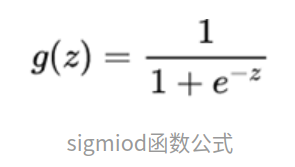蓝色线是sigmoid函数；红色线是sigmoid的求导；
优点：平滑、易于求导。 缺点： ①梯度的消失；饱和区域变化的很慢，趋近于０ ②输出的均值不为０，当输出大于0时，则梯度方向将大于0，也就是说接下来的反向运算中将会持续正向更新； ③幂函数还是比较难算的;计算量大 ④Sigmoid函数并不是以（0,0）为中心点
import numpy as np
import matplotlib.pyplot as plt
def sigmoid(x):
return 1.0/(1+np.exp(-x))

sigmoid_inputs = np.arange(-10,10,0.1)
sigmoid_outputs = sigmoid(sigmoid_inputs)
print("Sigmoid Function Input :: {}".format(sigmoid_inputs))
print("Sigmoid Function Output :: {}".format(sigmoid_outputs))

plt.plot(sigmoid_inputs,sigmoid_outputs)
plt.xlabel("Sigmoid Inputs")
plt.ylabel("Sigmoid Outputs")
plt.show()

2. tanh
tanh为双切正切曲线，过（0,0）点。相比Sigmoid函数，更倾向于用tanh函数； 公式为：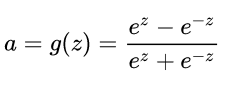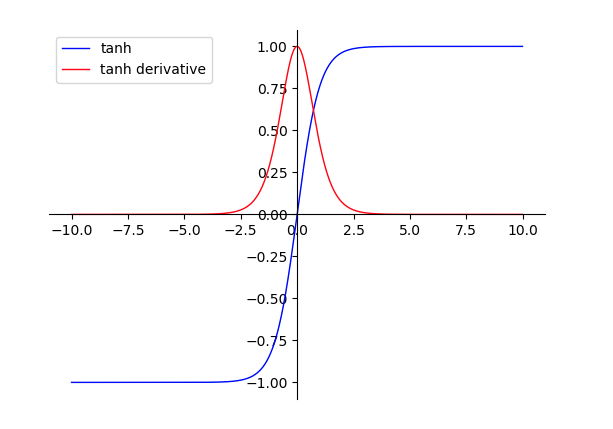蓝色线是sigmoid函数；红色线是tahn的求导； 优点： ①函数输出以（0,0）为中心 ②收敛速度相对于Sigmoid更快
缺点： ①梯度的消失；饱和区域变化的很慢，趋近于０；tanh并没有解决sigmoid梯度消失的问题 ②幂函数还是比较难算的;计算量大
目前比较多的使用的
1. RELU && RELU6
线性整流函数（Rectified Linear Unit, ReLU），又称修正线性单元；最近这几年很常用的激活函数。公式：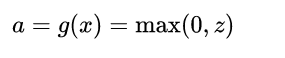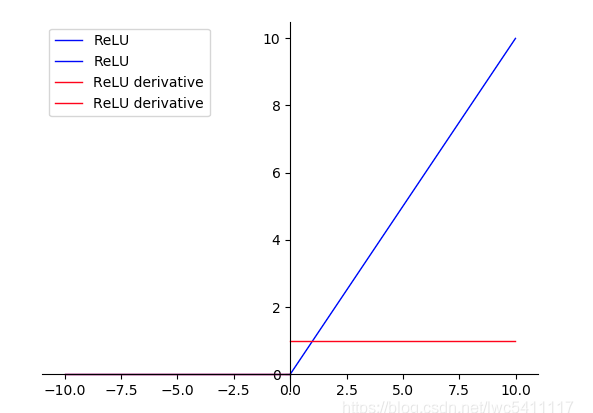蓝色线是RELU函数；红色线是RELU的求导；如上图，是不是贼简单，AlexNet的论文对ReLu和普通Sigmoid系函数做的对比测试，可以看到，ReLu的使用，使得学习周期大大缩短。综合速率和效率，DL中大部分激活函数应该选择ReLu。
大大缩短。
优点：
RELU中收敛速度要比Sigmoid和tanh快很多；计算量小啊，它不快谁快？更加有效率的梯度下降以及反向传播：避免了梯度爆炸和梯度消失问题对神经网络可以使用稀疏表达; 仿生物学原理：相关大脑方面的研究表明生物神经元的信息编码通常是比较分散及稀疏的(有兴趣再看吧（⊙ｏ⊙）)。
缺点：
在训练过程中容易出现神经元死亡，之后梯度永远为0的情况。在mobilenetv2系列中就分析了这玩意；
整体下来大家用了都说好！统治了很久的激活领域的top； 由relu为开始，PReLU，Leaky ReLU，CReLU等等各种变种相继登场；
relu6很好理解： 下图是relu6和relu之间的关系；主要是为了在移动端float16的低精度的时候，也能有很好的数值分辨率，如果对ReLu的输出值不加限制，那么输出范围就是0到正无穷，而低精度的float16无法精确描述其数值，带来精度损失。如果你是做移动端的，应该会很熟悉；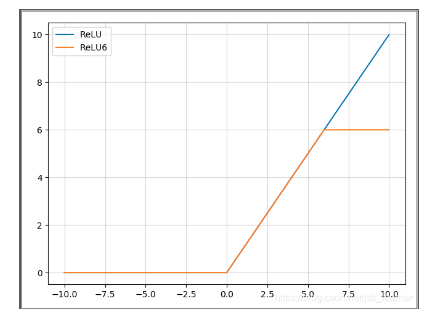2.Mish
Mish: A Self Regularized Non-Monotonic Neural Activation Function
一种自正则的非单调神经激活函数，平滑的激活函数允许更好的信息深入神经网络，从而得到更好的准确性和泛化。论文中提出，相比Swish有0.494%的提升，相比ReLU有1.671%的提升；该方法也在yolov4中得到了验证；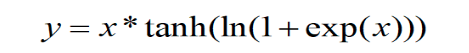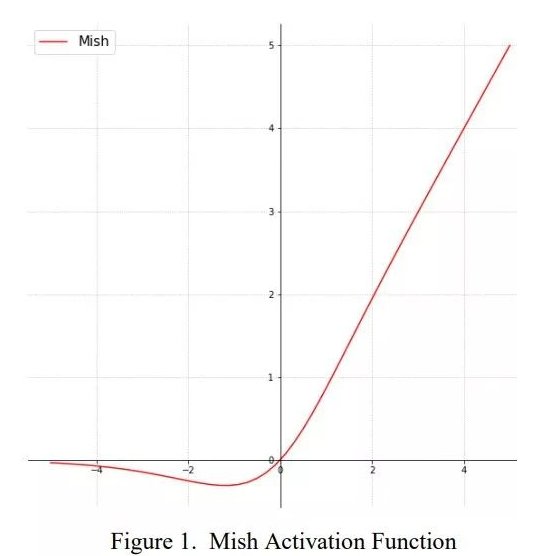这个曲线好像完美的模拟了生物那方面的什么曲线；…
import torch
import torch.nn as nn
import torch.nn.functional as F

class Mish(nn.Module):
def __init__(self):
super().__init__()
def forward(self,x):
x = x * (torch.tanh(F.softplus(x)))
return x

一开始没抱有太大希望，用了RELU6和ＭISH（自己目标检测的任务，非公开数据集）一对比；提升还是很明显的；博客也对MISH稳定性和精确度进行了多种任务的对比；整体下来，大多数任务都有了相应的提升；
激活函数RELU6ＭISHcoco得分0.49760.5214
优点：
以上无边界(即正值可以达到任何高度)避免了由于封顶而导致的饱和。理论上对负值的轻微允许允许更好的梯度流，而不是像ReLU中那样的硬零边界。平滑的激活函数允许更好的信息深入神经网络，从而得到更好的准确性和泛化。这个曲线好像完美的模拟了生物那方面的什么曲线； (有兴趣再看吧（⊙ｏ⊙）)。
缺点：
计算量肯定比relu大，占用的内存也多了不少；
3.Swish
（待验证，todo，写不动了）
Ohter
1. Leaky ReLU
公式：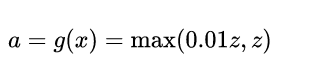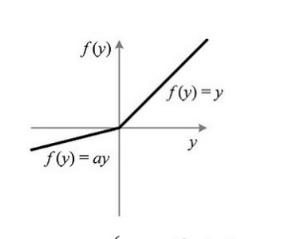# 兄弟们，自己用吧
torch.nn.LeakyReLU(negative_slope: float = 0.01, inplace: bool = False)

SELU
（待验证，todo，写不动了）
展开全文深度学习 激活函数 mish
• 最后发现，在较小的数据集中（大数据集未必），Leaky ReLU及其变体(PReLU、RReLU)的性能都要优于ReLU激活函数；而RReLU由于具有良好的训练随机性，可以很好的防止过拟合。 一、背景 我们在设计神经网络时候，在选择...PReLU RReLU 非饱和激活函数
• 1.2、大脑工作原理：稀疏性原理1.3、稀疏性优点二、ReLU定义三、ReLU函数优缺点3.1、优点3.2、缺点四、几个其他变体激活函数4.1、Leaky ReLU4.2、PReLU(parametric ReLU)4.3、RReLU(Random ReLU)4.4、ReLU6(抑制其...激活函数
• SELUs - 以可视化和直方图在ReLU和Leaky ReLU之间进行比较
• Understanding Training Dynamics of Deep ReLU Networks
• 文章目录一、前言二、拟解决的关键问题三、Dynamic ReLU1. 激活函数2. Dynamic ReLU3. Dynamic ReLU的实现四、总结参考文献 一、前言 论文地址：https://arxiv.org/abs/2003.10027 这篇博客主要是对Dynamic ReLU这篇...深度学习 神经网络 分类算法
• 本篇博文主要来源于对文章 Unwrapping The Black Box of Deep ReLU Networks: Interpretability, Diagnostics, and Simplification 的学习，同时还有部分内容摘自wiki百科。 什么是ReLU函数？ ReLU，全称为：...可解释性 深度学习 人工智能 神经网络
• ReLU到GELU，一文概览神经网络的激活函数（https://mp.weixin.qq.com/s/pA9JW75p9J5e5KHe3ifcBQ），除上述外，本文还提供了若干相应的支撑论文。
• 1.22.1.ReLU torch.nn.ReLU() 1.22.2.RReLU torch.nn.RReLU() 1.22.3.LeakyReLU torch.nn.LeakyReLU() 1.22.4.PReLU torch.nn.PReLU() 1.22.5.Sofplus torch.nn.Softplus() 1.22.6.ELU torch.nn.EL
• ## ReLu

千次阅读 2018-03-05 11:05:28
ReLu是神经网络中的一个激活函数，其优于tanh和sigmoid函数。1.为何引入非线性的激活函数？如果不用激活函数，在这种情况下每一层输出都是上层输入的线性函数。容易验证，无论神经网络有多少层，输出都是输入的线性...激活函数...